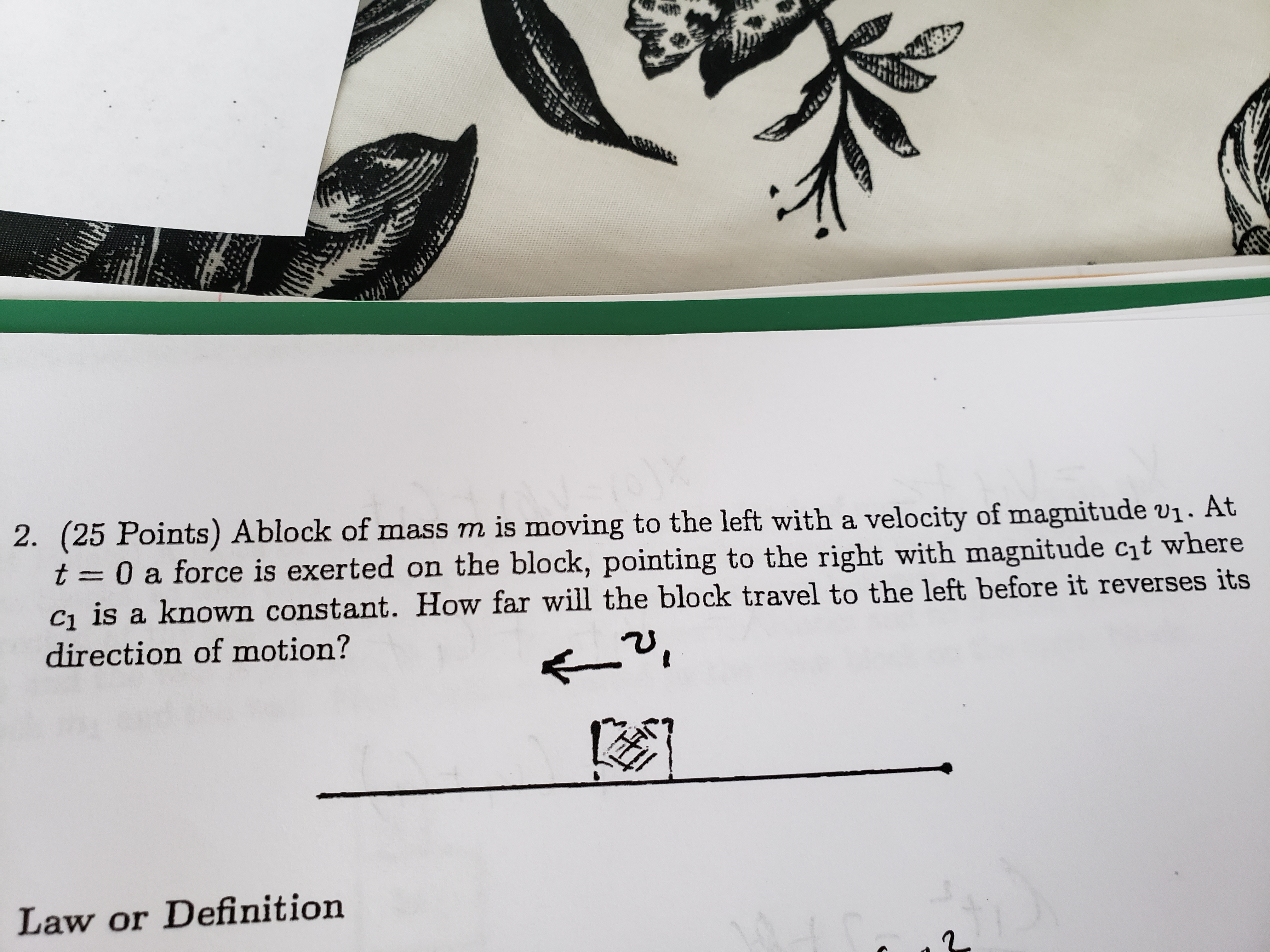# 2. (25 Points) Ablock of mass m is moving to the left with a velocity of magnitude vi. Att 0 a force is exerted on the block, pointing to the right with magnitude cht whereci is a known constant. How far will the block travel to the left before it reverses itsdirection of motion?Law or Definition

Question
42 viewshelp_outlineImage Transcriptionclose2. (25 Points) A block of mass m is moving to the left with a velocity of magnitude vi. At t 0 a force is exerted on the block, pointing to the right with magnitude cht where ci is a known constant. How far will the block travel to the left before it reverses its direction of motion? Law or Definition fullscreen
check_circle

Step 1

Starting from the expression for acceleration,

Step 2

At the point where the direction of the mass is reversed, v = 0. Therefore,

Step 3

The distance travelled by the block before r...

### Want to see the full answer?

See Solution

#### Want to see this answer and more?

Solutions are written by subject experts who are available 24/7. Questions are typically answered within 1 hour.*

See Solution
*Response times may vary by subject and question.
Tagged in

### Kinematics